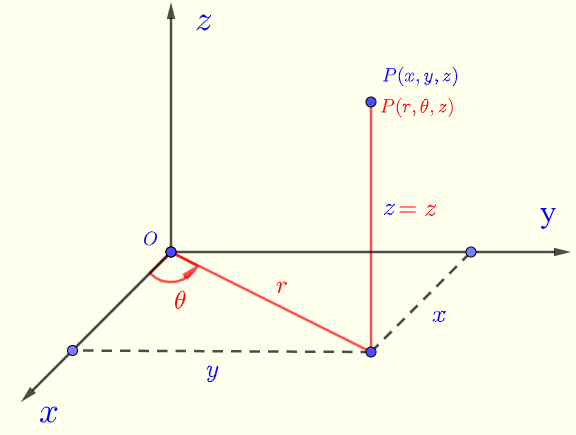# Convert Cylindrical to Rectangular Coordinates - Calculator

## Cylindrical and Rectangular Coordinates

Convert rectangular to spherical coordinates using a calculator.
Using trigonometric ratios, it can be shown that the cylindrical coordinates $(r,\theta,z)$ and rectangular coordinates $(x,y,z)$ in Fig.1 are related as follows:
$x = r \cos \theta$ , $y = r \sin \theta$ , $z = z$       (I)
$r = \sqrt {x^2 + y^2}$ , $\tan \theta = \dfrac{y}{x}$ , $z = z$       (II)
with $0 \le \theta \lt 2\pi$Fig.1 - Cylindrical and Rectangular coordinates The calculator calculates the rectangular coordinates $x$ , $y$ and $z$ given the cylindrical coordinates $r$ , $\theta$ and $z$ using the formulas in I above.

## Use Calculator to Convert Cylindrical to Rectangular Coordinates

1 - Enter $r$, $\theta$ and $z$ and press the button "Convert". You may also change the number of decimal places as needed; it has to be a positive integer.
Angle $\theta$ may be entered in radians and degrees.

 $r =$ 2 $\theta =$ 45 degrees radians $z =$ = 5 Number of Decimal Places = 5 $x =$ $y =$ $z =$

## More References and links

Maths Calculators and Solvers.